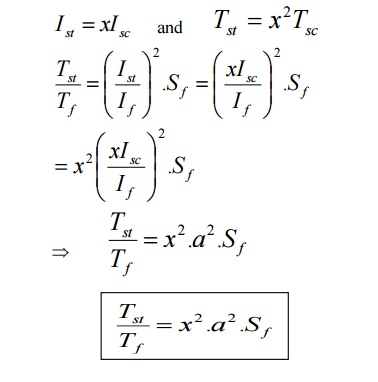Home | | Electrical Drives and Controls | Stator Resistance (or) Primary Resistance Starter

# Stator Resistance (or) Primary Resistance Starter

Their purpose is to drop some voltage and hence reduce the voltage applied across the motor terminals.

STATOR RESISTANCE (OR) PRIMARY RESISTANCE STARTER

Reduced voltage starting:

Their purpose is to drop some voltage and hence reduce the voltage applied across the motor terminals.

In this way, the initial current drawn by the motor is reduced.

However, it should be noted that whereas current varies directly as the voltage, the torque varies as square of applied voltage.

[Note: When applied voltage is reduced, the rotating flux f is reduced which in turn decreases rotor e.m.f and hence rotor current I2. Starting torque which depends both on f and I2 suffers on two counts when impressed voltage is reduced]

For example if voltage applied across motor terminals is reduced by 50%, starting current is reduced by 50%, but torque is reduced to 25% of the full-voltage value.When motor is direct-switched on to normal voltage, then starting current is the short –circuit current Isc.Starting torque = 1.96 x Full load torque.

Hence, even if current is greater than full load current the starting torque is only 1.96 times full-load torque.

If applied voltages/phase can be reduced by fraction „x‟, thenIt is obvious that the ratio of starting torque of full-load torque is x2 of that obtained with direct switching (or) across the line starting. This method is useful for the smooth starting of small machines only.

Study Material, Lecturing Notes, Assignment, Reference, Wiki description explanation, brief detail
Electrical Drives & Control : Starting Methods : Stator Resistance (or) Primary Resistance Starter |